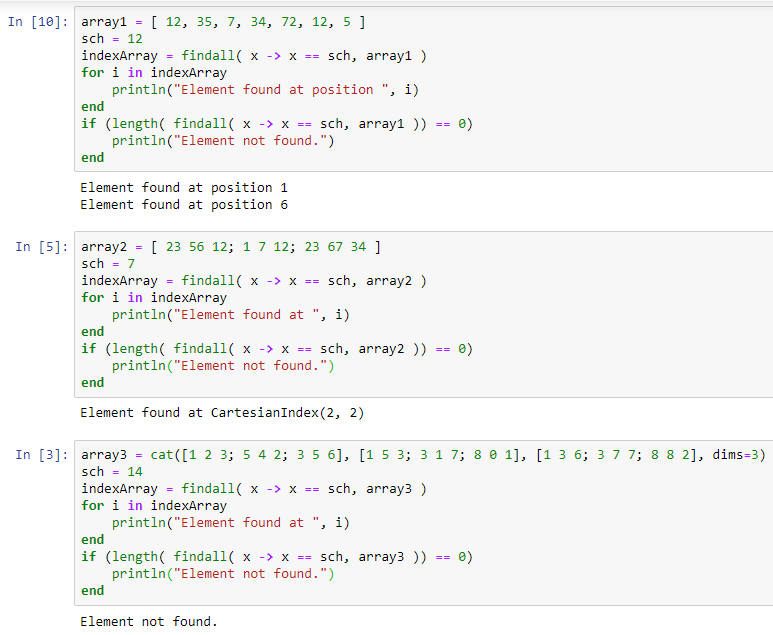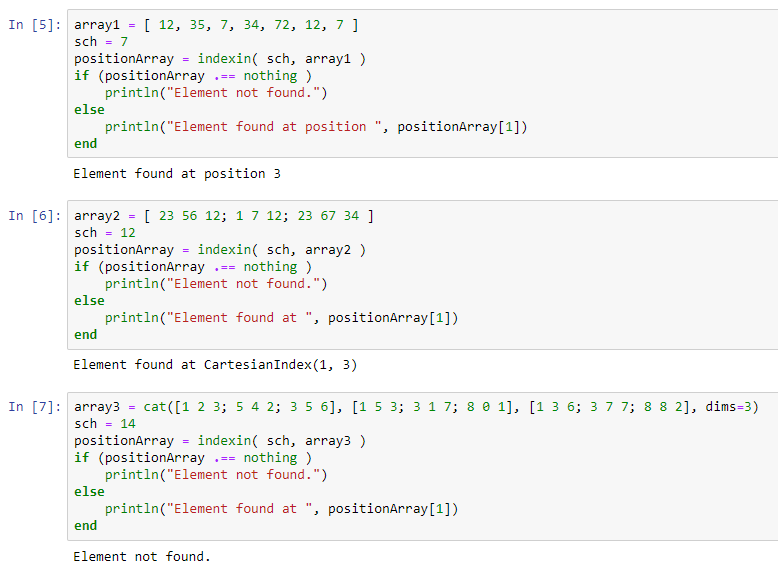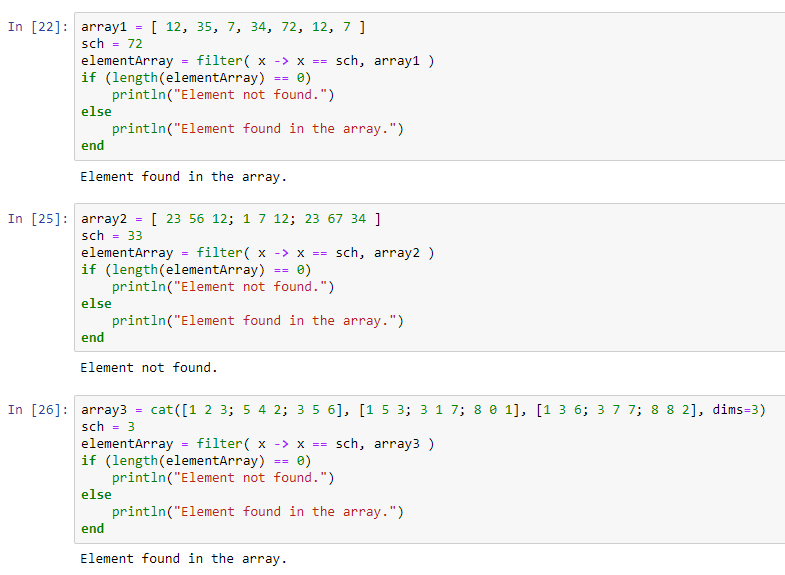# Searching in Array for a given element in Julia

Given an array (1D, 2D or 3D), and an element to look for in the array. If the element is present in the array, then print the position of the element in the array, else print “Element not found”.
In the recent updates to Julia, the developers have unified the `search()` and `find()` functions into a simpler `findall()` function. However, the rest of its counterparts, such as, `findnext()`, `findprev()` etc. are still accessible. The `find()` function is replaced by `filter()` method, and a new `indexin()` function is added which returns the index of particular elements from an array.

Examples:

```Input : array1 = [ 5, 9, 1, 8, 2, 7 ]
sch = 8
Output : Element found at position 4

Input : array2 = [ 7 9 2
9 4 1
8 4 6 ]
sch = 4
Output : Element found at CartesianIndex(2, 2).
Element found at CartesianIndex(3, 2).

Input : array3 = [ 22, 7, 91, 69, 2, 74 ]
sch = 6

[Note: Unlike other languages, In Julia indexing of an Array starts with 1.]
```

### Using `findall()` method

The `findall()` method is used to find all the occurrences of the required element from an array, and return an Array (or CartesianArray, depending on the dimensions of input array) of the index, where the elements are present in the input array. If the element is not present in the input array, it returns an empty array.
Syntax:

`findall(::Function, ::Number)`

 `# Julia program to illustrate the  ` `# use of findall() method to search  ` `# for an element in an array. ` `  `  `# Searching for an element in a 1D ARRAY ` `array1 ``=` `[ ``12``, ``35``, ``7``, ``34``, ``72``, ``12``, ``5` `] ` `sch ``=` `12` `  `  `indexArray ``=` `findall( x ``-``> x ``=``=` `sch, array1 ) ` `for` `i ``in` `indexArray ` `    ``println(``"Element found at position "``, i) ` `end ` `if` `(length( findall( x ``-``> x ``=``=` `sch, array1 )) ``=``=` `0``) ` `    ``println(``"Element not found."``) ` `end ` `  `  `# Searching for an element in a 2D ARRAY ` `# of shape (3 x 3). ` `array2 ``=` `[ ``23` `56` `12``; ``1` `7` `12``; ``23` `67` `34` `] ` `sch ``=` `7` `  `  `indexArray ``=` `findall( x ``-``> x ``=``=` `sch, array2 ) ` `for` `i ``in` `indexArray ` `    ``println(``"Element found at "``, i) ` `end ` `if` `(length( findall( x ``-``> x ``=``=` `sch, array2 )) ``=``=` `0``) ` `    ``println(``"Element not found."``) ` `end ` `   `  `# Searching for an element in a 3D ARRAY ` `# of shape (3 x 3 x 3). ` `array3 ``=` `cat([``1` `2` `3``; ``5` `4` `2``; ``3` `5` `6``], ` `             ``[``1` `5` `3``; ``3` `1` `7``; ``8` `0` `1``], ` `             ``[``1` `3` `6``; ``3` `7` `7``; ``8` `8` `2``], dims``=``3``) ` `sch ``=` `14` `  `  `indexArray ``=` `findall( x ``-``> x ``=``=` `sch, array3 ) ` `for` `i ``in` `indexArray ` `    ``println(``"Element found at "``, i) ` `end ` `if` `(length( findall( x ``-``> x ``=``=` `sch, array3 )) ``=``=` `0``) ` `    ``println(``"Element not found."``) ` `end `

Output:### Using `indexin()` method

The `indexin()` function is used to search for the position of the first occurrence of the element in the array (searches row-wise) and return its position in the array. However, the search stops as soon as the searched element is found in the array. Thus, this function always returns an array of length 1. If the searched element is not found in the array, it returns `nothing` in an array.
Syntax:

`indexin(::Any, ::AbstractArray)`

 `# Julia program to illustrate the  ` `# use of indexin() method to search  ` `# for an element in an array. ` `  `  `# Searching for an element in a 1D ARRAY ` `array1 ``=` `[ ``12``, ``35``, ``7``, ``34``, ``72``, ``12``, ``7` `] ` `sch ``=` `7` `  `  `positionArray ``=` `indexin( sch, array1 ) ` `if` `(positionArray .``=``=` `nothing ) ` `    ``println(``"Element not found."``) ` `else` `    ``println(``"Element found at position "``,  ` `             ``positionArray[``1``]) ` `end ` `  `  `# Searching for an element in a 2D ARRAY ` `# of shape (3 x 3). ` `array2 ``=` `[ ``23` `56` `12``; ``1` `7` `12``; ``23` `67` `34` `] ` `sch ``=` `12` `  `  `positionArray ``=` `indexin( sch, array2 ) ` `if` `(positionArray .``=``=` `nothing ) ` `    ``println(``"Element not found."``) ` `else` `    ``println(``"Element found at "``, positionArray[``1``]) ` `end ` `  `  `# Searching for an element in a 3D ARRAY ` `# of shape (3 x 3 x 3). ` `array3 ``=` `cat([``1` `2` `3``; ``5` `4` `2``; ``3` `5` `6``], ` `             ``[``1` `5` `3``; ``3` `1` `7``; ``8` `0` `1``], ` `             ``[``1` `3` `6``; ``3` `7` `7``; ``8` `8` `2``], dims``=``3``) ` `sch ``=` `14` `  `  `positionArray ``=` `indexin( sch, array3 ) ` `if` `(positionArray .``=``=` `nothing ) ` `    ``println(``"Element not found."``) ` `else` `    ``println(``"Element found at "``, positionArray[``1``]) ` `end `

Output:### Using `filter()` method

The `filter()` function is generally used to filter elements from the array that are satisfying some particular condition. But, we can also use this function to search for elements in an array. However, this function, instead of returning index, returns the elements itself from the array. So, we could just check the size of the array returned by this function, and print accordingly.
Syntax:

`filter(::Any, ::Array{T, N}) where {T, N} at array.`

 `# Julia program to illustrate the  ` `# use of filter() method to search  ` `# for an element in an array. ` `  `  `# Searching for an element in a 1D ARRAY ` `array1 ``=` `[ ``12``, ``35``, ``7``, ``34``, ``72``, ``12``, ``7` `] ` `sch ``=` `72` `  `  `elementArray ``=` `filter``( x ``-``> x ``=``=` `sch, array1 ) ` `if` `(length(elementArray) ``=``=` `0``) ` `    ``println(``"Element not found."``) ` `else` `    ``println(``"Element found in the array."``) ` `end ` `  `  `# Searching for an element in a 2D ARRAY ` `# of shape (3 x 3). ` `array2 ``=` `[ ``23` `56` `12``; ``1` `7` `12``; ``23` `67` `34` `] ` `sch ``=` `33` `  `  `elementArray ``=` `filter``( x ``-``> x ``=``=` `sch, array2 ) ` `if` `(length(elementArray) ``=``=` `0``) ` `    ``println(``"Element not found."``) ` `else` `    ``println(``"Element found in the array."``) ` `end ` `  `  `# Searching for an element in a 3D ARRAY ` `# of shape (3 x 3 x 3). ` `array3 ``=` `cat([``1` `2` `3``; ``5` `4` `2``; ``3` `5` `6``], ` `             ``[``1` `5` `3``; ``3` `1` `7``; ``8` `0` `1``],  ` `             ``[``1` `3` `6``; ``3` `7` `7``; ``8` `8` `2``], dims``=``3``) ` `sch ``=` `3` `  `  `elementArray ``=` `filter``( x ``-``> x ``=``=` `sch, array3 ) ` `if` `(length(elementArray) ``=``=` `0``) ` `    ``println(``"Element not found."``) ` `else` `    ``println(``"Element found in the array."``) ` `end `

Output:Apart from all these inbuilt functions for searching an element in array, we could also use the two most common searching algorithms, i.e. Linear Search and Binary search.

My Personal Notes arrow_drop_upCheck out this Author's contributed articles.

If you like GeeksforGeeks and would like to contribute, you can also write an article using contribute.geeksforgeeks.org or mail your article to contribute@geeksforgeeks.org. See your article appearing on the GeeksforGeeks main page and help other Geeks.

Please Improve this article if you find anything incorrect by clicking on the "Improve Article" button below.

Article Tags :

1

Please write to us at contribute@geeksforgeeks.org to report any issue with the above content.